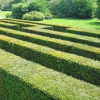#### You may also likeThis comes in two parts, with the first being less fiendish than the second. Itâ€™s great for practising both quadratics and laws of indices, and you can get a lot from making sure that you find all the solutions. For a real challenge (requiring a bit more knowledge), you could consider finding the complex solutions.### Discriminating

You're invited to decide whether statements about the number of solutions of a quadratic equation are always, sometimes or never true.This will encourage you to think about whether all quadratics can be factorised and to develop a better understanding of the effect that changing the coefficients has on the factorised form.

# Proving the Laws of Logarithms

##### Age 16 to 18Challenge LevelThis resource is from Underground Mathematics.

Warm up

Take a look at these results

$$\log_3 2 + \log_3 5 = \log_3 10$$
$$\log_2 15 - \log_2 3 = \log_2 5$$
$$2\log_5 7 = \log_5 49$$
$$\frac{1}{3} \log_5 64 = \log_5 4$$
$$(\log_5 7) \times (\log_7 11)=\log_5 11$$

How can you change the input values so that the equations still hold?

What happens if you change the base of the logarithms?

Can you state generalised versions of these results?  What conditions must the base and the inputs satisfy?

Main problem

These cards can be sorted to give a proof of the statement

$\log_c a + \log_c b = \log_c ab$ for any $a,b > 0$ and $c>0$, but $c \neq 1$.

Can you arrange the cards to give a convincing proof?

You may need to include some additional algebraic steps or explanations if you think they would help to make the argument clearer or more convincing.

You might want to print out the cards and rearrange them. Some blank cards have been included in the cards for printing in case you would like to use them to fill in some details.

One challenge we face when trying to prove something is making it clear what our proof is building on. Our starting point here is that we know how to manipulate indices or powers and we know a relationship between indices and logarithms.  We will use results about manipulating indices to prove a result about manipulating logarithms.

This is an Underground Mathematics resource.

Underground Mathematics is hosted by Cambridge Mathematics. The project was originally funded by a grant from the UK Department for Education to provide free web-based resources that support the teaching and learning of post-16 mathematics.

Visit the site at undergroundmathematics.org to find more resources, which also offer suggestions, solutions and teacher notes to help with their use in the classroom.